Next: Matrix Eigenvalue Theory Up: Rigid Body Rotation Previous: Moment of Inertia Tensor

# Rotational Kinetic Energy

The instantaneous rotational kinetic energy of a rotating rigid body is written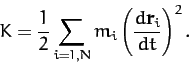(467)

Making use of Equation (457), and some vector identities (see Section A.9), the kinetic energy takes the form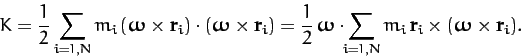(468)

Hence, it follows from (458) that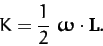(469)

Making use of Equation (466), we can also write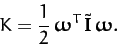(470)

Here,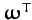is the row vector of the Cartesian components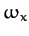,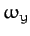,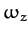, which is, of course, the transpose (denoted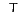) of the column vector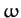. When written in component form, the above equation yields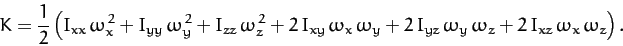(471)

Richard Fitzpatrick 2011-03-31